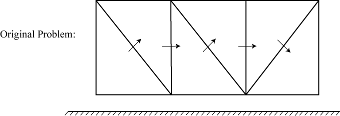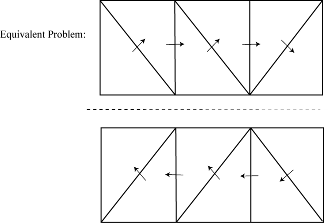## Infinite Ground Plane

Antenna Toolbox™ library element uses the image theory technique to model an infinite ground plane. The main advantage of image theory technique is that you do not need to discretize the ground plane. Image theory reduces the overall size of the problem and you get a solution faster.

### Image Theory

The image theory technique uses a catalog of electromagnetic problems that produce identical field distributions. You can identify these electromagnetic problems by noting that conducting surfaces are of constant potential. Placing these conducting surfaces along any equipotential lines in any field distributions does not alter the fields.

Consider a positive and negative charge placed a distance of 2x apart from each other. The equipotential surface for these two charges forms at a distance midway between them.If you place a conducting object along this equipotential surface, then the field above the surface does not change.You can also apply this equivalence in reverse. Consider charges that are distance x apart from a conducting surface. You can replace the conducting surface with a set of image charges whose signs are opposite those of the original charge. Place these image charges at a distance x below the original conducting surface. This method eliminates the conducting plate, leaving only charges in unbound space. This equivalence is called the method of images.

### Formulate the Image Theory Technique

Consider a rectangular plate over an infinite ground plane. This rectangular plate is divided into a triangular mesh. The induced currents flow over the triangulated surface using the RWG basis functions.You formulate the problem by considering real and image basis functions. You can show the same problem using image theory or method of images. The interaction matrix is calculated using the interaction between real and image basis functions.According to the method of images, the real and image electric currents are equal to each other in terms of magnitude. The parallel components are in opposite direction. The normal components are in the same direction.Balanis, C.A. Antenna Theory. Analysis and Design, 3rd Ed. New York: Wiley, 2005.

 Yildrium, Cemal. "Scattering and Radiation problems of arbitrary shaped conducting bodies above ground plane" Ph.D. Thesis. Bilkent University, Ankara, Turkey, October, 2002